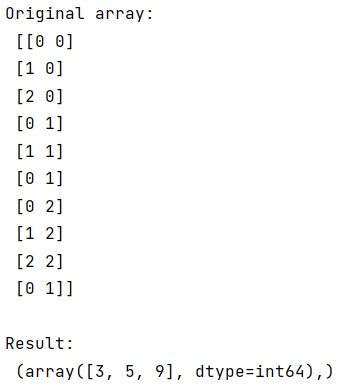# Find matching rows in 2 dimensional NumPy array

Learn how to find matching rows in 2 dimensional NumPy array in Python?
Submitted by Pranit Sharma, on February 11, 2023

NumPy is an abbreviated form of Numerical Python. It is used for different types of scientific operations in python. Numpy is a vast library in python which is used for almost every kind of scientific or mathematical operation. It is itself an array which is a collection of various methods and functions for processing the arrays.

## NumPy Array - Finding Matching Rows in 2 Dimensional

Suppose that we are given a numpy array that contains multiple rows and some of them are matching rows and we need to find the index of this 2-dimensional array that matches a row.

For this purpose, we need the numpy.where() method to get the indexes. We will use numpy.where() and pass a certain condition that where ever the array is equal to a specific row value, we need to return its index and also we will do this for all rows so we will also apply all() with it.

Let us understand with the help of an example,

## Python code to find matching rows in 2 dimensional NumPy array

```# Import numpy
import numpy as np

# Creating a numpy array
arr =  np.array([[0, 0],[1, 0],[2, 0],[0, 1],[1, 1],[0, 1],[0, 2],[1, 2],[2, 2],[0, 1]])

# Display original array
print("Original array:\n",arr,"\n")

# returning indices of matching rows
res = np.where((arr == (0, 1)).all(axis=1))

# Display result
print("Result:\n",res)
```

Output: